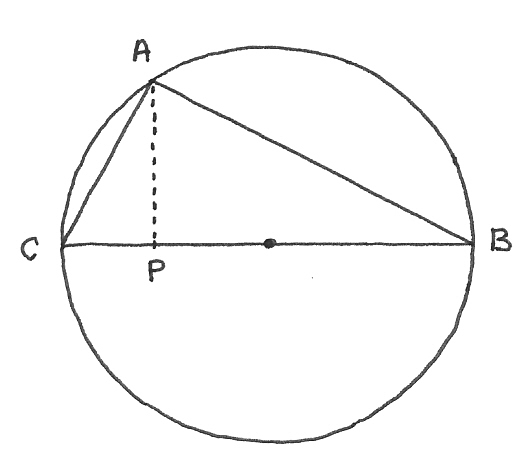# »  Solutions to puzzles in my National Review Online Diary

##May 2005

—————————

In my May diary I posed the following brain-teaser.

For ten years a teacher exposed his student to the following test:

A right-angle triangle has hypoteneuse 10 inches, and the height drawn from the right angle to the hypoteneuse is 6 inches. Find the area of this right-angle triangle.
For 10 years the students satisfied their teacher with the following answer:  Area = (10 × 6) / 2 = 30 square inches.

Then at the eleventh year a smart student came, who could not solve this problem. Why?

—————————Solution

Data (i.e. the things given):  Angle at A a right angle, AP perpendicular to BC, BC = 10, AP = 6.

Quaesita (the things sought): Area of the triangle ABC.

Naive approach (wrong):  Area of a triangle is half the base times the height. In this case the base is 10, the height is 6, so the area is 30 square units.

Smart approach (correct):  Construct a circle with BC as diameter. Plainly the radius of this circle is 5.

By a well-known theorem of plane geometry (Euclid's III.31), if the angle at A is to be a right angle, then A must lie on the perimeter of the circle.

It follows that the maximum possible value of AP is 5.

The triangle as given is therefore an impossible object, and the problem has no solution.